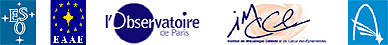# The Venus Transit 2004

## ... Extended InfoSheet G3

Printable Page: html       PDF

# The definition of the Astronomical Unit and the other units of distance in astronomy

The unit of length in the international system (SI) is the metre, defined as follows: the metre is the distance traversed by light in the vacuum in the time interval of 1/299 792 458 of a second.

In astronmy, the basic unit of distance is the "Astronomical Unit (AU)" , defined as follows: the radius of the circular orbit of a planet of negligible mass and free of perturbations, whose mean motion isl "k" radians per day around the Sun, where "k" is Gauss' gravitational constant, the unit of time being the solar day and the unit of mass, the mass of the Sun.

The basic unit in astronomy is thus linked to the dimensions of the solar system and to the motion of the Earth. Indeed, all distances in the solar system may be deduced from only one of them, thanks to Kepler's laws. The perturbations generated by the Moon and the other planets on the Earth, are known only through dynamic modelling of the solar system. The problems generated by these poorly understood perturbations can be avoided by using a fictitious planet having simple circular motion which is connected to the model of the solar system.

To define the Astronomical Unit using the real Earth would require a complete understanding of the Earth-Moon system.

We now have to evaluate the Astronomical Unit in terms of the SI unit of length, the metre. This is done by calculating parallaxes or by using radar reflections from nearby planets. The use of the Astronomical Unit makes it possible to preserve the same numerical quantities in modelling the solar system, even if the value of the Astronomical Unit itself changes and is improved by increasingly precise measurements.

The unit derived from the Astronomical Unit is the "solar parallax" which defined as follows: the solar parallax is the arc sine of the terrestrial radius divided by the astronomical unit.

There are auxiliary units of length defined as follows:

• a parsec is the distance of a star whose annual parallax is equal to one arc second, i.e. the distance at which one astronomical unit subtends an angle of one second of arc;
• a light-year is the distance travelled by light during one Julian year (365.25 days) in empty space.

Only the metre corresponds to an arbitrary defined constant; the other units are derived and their values can vary with time. Gauss' gravitational constant has as a value (UAI, 1976 and IERS, 1992) of 0.017 202 098 95 radian per day, that is to say 0.985 607 668 601 425 degree per day.

The following table give the current values of these constants.

 Solar parallax Astronomical unit Symbol π 0 AU Unit Arc second Metre Newcomb 1895 8,790 Paris 1896 8.80 De Sitter 1938 8.8030 149 453 000 000 Clemence 1948 8.790 149 670 000 000 UAI 1964 8.79405 149 600 000 000 UAI 1976 8.794148 149 597 870 000 JPL DE200 1982 149 597 870 660 IERS 1992 8.794142 149 597 870 610 JPL DE403 1995 149 597 870 691

Finally, for a value of the astronomical unit corresponding to IERS (1992) i.e. 149 597 870 610 metres, the auxiliary units of length are :

• 1 light-year = 63,241.077 10 au
• 1 parsec = 206,264.806 248 au

Go to the corresponding Brief InfoSheet

Back to the List of Extended InfoSheets.